# 5G/NR - CSI RS

CSI RS

As in LTE CSI, NR CSI (Channel Status Information) is a mechanism that a UE measure various radio channel quality and report the result to Network(gNB).

There are pretty complicated factors involved in CSI operation, but this page would focus on CSI signal generation and Resource Mapping. Other CSI operation procedure will be explained in other page.

For most of LTE case, we don't need any special signal for CSI since we used Cell Specific Reference Signal. However, from LTE TM9 we stared using a special reference signal for CSI. So if you have some understandings on LTE CSI Reference signal in TM9 or higher, it would be relatively easier for you to understand this page even if NR CSI RS(Reference Signal) is constructed more complicated way comparing to LTE CSI RS.

Sequence Generation and Resource Mapping

As you may notice in the following equation, NR CSI is based on Pseudo Random Sequence. Then this sequence is multipied by sepcially designed weighting sequence in both time domain and frequency domain and than scaled by power scaling factor. And then this sequence is mapped to a set of specific resource elements in Resource Grid. All of these process can be summarized as follows and so many factors are involved in this procedure.

If you are not the physical layer developer who need to implement this part, you don't need to go through this to the full details, but it will be beneficial to pay attention to various parameters (especially RRC parameters) involved in this process.Tables for Resource Element Mapping

< 38.211-Table 7.4.1.5.3-1: CSI-RS locations within a slot. >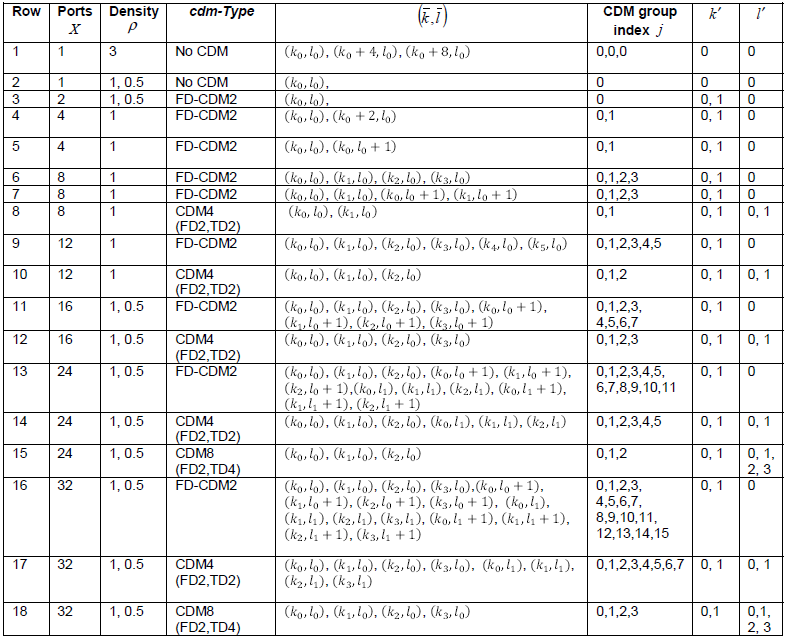The reference location of CSI-RS in time domain is determined by RRC layer as below.==

The reference location of CSI-RS in frequency domain (k1,k2,k3) is determined by RRC layer as below

••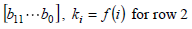•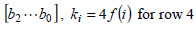•Ports, Density, cdm-Type are specified by following RRC parameters

Tables for CDM Sequence Generation

CDM table is used for CSI-RS signal generation as shown below.Each element values of CDM tables are specified in 38.211 as shown below.

< 38.211 - Table 7.4.1.5.3-2: The sequences wf(k) f and wt(l) for cdm-Type equal to 'no CDM'. >< 38.211 - Table 7.4.1.5.3-3: The sequences wf(k) f and wt(l) for cdm-Type equal to 'FD-CDM2'. >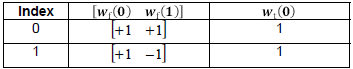< 38.211 - Table 7.4.1.5.3-4: The sequences wf(k) f and wt(l) for cdm-Type equal to 'CDM4'. >< 38.211 - Table 7.4.1.5.3-5: The sequences wf(k) f and wt(l) for cdm-Type equal to 'CDM8'. >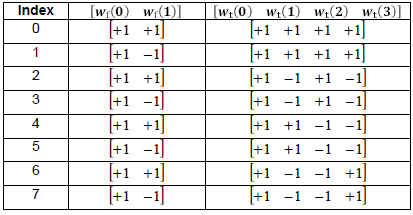RRC Parameter : NZP-CSI-RS-Resource

Almost all of the contents in this page is about the IE(Information Element) resourceMapping. The IE resourceMapping is a part of the NZP-CSI-RS-Resource as shown below.

NZP-CSI-RS-Resource ::= SEQUENCE {

nzp-CSI-RS-ResourceId      NZP-CSI-RS-ResourceId,

resourceMapping            CSI-RS-ResourceMapping,

powerControlOffset         INTEGER (-8..15),

powerControlOffsetSS       ENUMERATED{db-3, db0, db3, db6} OPTIONAL, -- Need R

scramblingID               ScramblingId,

periodicityAndOffset       CSI-ResourcePeriodicityAndOffset OPTIONAL,-

qcl-InfoPeriodicCSI-RS     TCI-StateId OPTIONAL, -- Cond Periodic

...

}

resourceMapping : Refer to the section CSI-RS-ResourceMapping for the details.

powerControlOffset : Power offset of PDSCH RE to NZP CSI-RS RE. Value in dB

powerControlOffsetSS : Power offset of NZP CSI-RS RE to SSS RE. Value in dB

qcl-InfoPeriodicCSI-RS : For a target periodic CSI-RS, contains a reference to one TCI-State in TCI-States for providing the QCL source and QCL type. For periodic CSI-RS, the source can be SSB or another periodic-CSI-RS. Refers to the TCI-State which has this value for tci-StateId and is defined in tci-StatesToAddModList in the PDSCH-Config included in the BWPDownlink corresponding to the serving cell and to the DL BWP to which the resource belongs to.

In short, this indicates the tci-StateId to which this CSI-RS is QCLed.

How UE figure out which CSI-RS the network is using ? (CSI-RS-ResourceMapping)

When Network allocate the CSI-RS, it selects a specific row from 38.211-Table 7.4.1.5.3-1 and fill in a set of resource elements with the specific signal. This part would be straightforward.. the challenging part is how UE can figure out which CSI-RS the gNB(Network) is using.

The answer is that Network informs UE of all the details of CSI-RS via RRC message. Let's look into what kind of RRC parameters get involved in this process.

How to determine CSI-RS port and cdm (in Row number of 38.211 Table 7.4.1.5.3-1) ?

: This is specified by nrofPorts and cdm-Type in CSI-RS-ResourceMapping as shown below.

CSI-RS-ResourceMapping ::= SEQUENCE {

frequencyDomainAllocation CHOICE {

row1     BIT STRING (SIZE (4)),

row2     BIT STRING (SIZE (12)),

row4     BIT STRING (SIZE (3)),

other    BIT STRING (SIZE (6))

},

nrofPorts                       ENUMERATED {p1,p2,p4,p8,p12,p16,p24,p32},

firstOFDMSymbolInTimeDomain     INTEGER (0..13),

firstOFDMSymbolInTimeDomain2    INTEGER (2..12) OPTIONAL, -- Need R

cdm-Type                        ENUMERATED {noCDM, fd-CDM2, cdm4-FD2-TD2, cdm8-FD2-TD4},

density CHOICE {

dot5      ENUMERATED {evenPRBs, oddPRBs},

one       NULL,

three     NULL,

spare     NULL

},

freqBand    CSI-FrequencyOccupation,

...

}

How to determine REs(Resource Elements) for the selected CSI-RS ?

: This is configured by (k_bar, l_bar) column and frequencyDomainAllocation and firstOFDMSymbolInTimeDomain of CSI-RS-ResourceMapping as shown below.CSI-RS-ResourceMapping ::= SEQUENCE {

frequencyDomainAllocation CHOICE {

row1     BIT STRING (SIZE (4)),

row2     BIT STRING (SIZE (12)),

row4     BIT STRING (SIZE (3)),

other    BIT STRING (SIZE (6))

},

nrofPorts                       ENUMERATED {p1,p2,p4,p8,p12,p16,p24,p32},

firstOFDMSymbolInTimeDomain     INTEGER (0..13),

firstOFDMSymbolInTimeDomain2    INTEGER (2..12) OPTIONAL, -- Need R

cdm-Type                        ENUMERATED {noCDM, fd-CDM2, cdm4-FD2-TD2, cdm8-FD2-TD4},

density CHOICE {

dot5      ENUMERATED {evenPRBs, oddPRBs},

one       NULL,

three     NULL,

spare     NULL

},

freqBand    CSI-FrequencyOccupation,

...

}

CSI-FrequencyOccupation ::= SEQUENCE {

startingRB    INTEGER (0..maxNrofPhysicalResourceBlocks-1),

nrofRBs       INTEGER (24..maxNrofPhysicalResourceBlocksPlus1),

...

}

startingRB : PRB where this CSI resource starts in relation to common resource block #0 (CRB#0) on the common resource block grid. Only multiples of 4 are allowed (0, 4, ...)

nrofRBs : Number of PRBs across which this CSI resource spans. Only multiples of 4 are allowed. The smallest configurable number is the minimum of 24 and the width of the associated BWP. If the configured value is larger than the width of the corresponding BWP, the UE shall assume that the actual CSI-RS bandwidth is equal to the width of the BWP.

Combining thse two, we come come up with an example as shown below.How to figure out what kind of (Logical) Antenna Configuration Network is using ?

: This is another complicated story and is explained in a separate page here.

CSI RS Resource Mapping Examples

This section is to show various examples of configuring following RRC Parameters.

CSI-RS-ResourceMapping ::= SEQUENCE {

frequencyDomainAllocation CHOICE {

row1     BIT STRING (SIZE (4)),

row2     BIT STRING (SIZE (12)),

row4     BIT STRING (SIZE (3)),

other    BIT STRING (SIZE (6))

},

nrofPorts                       ENUMERATED {p1,p2,p4,p8,p12,p16,p24,p32},

firstOFDMSymbolInTimeDomain     INTEGER (0..13),

firstOFDMSymbolInTimeDomain2    INTEGER (2..12) OPTIONAL, -- Need R

cdm-Type                        ENUMERATED {noCDM, fd-CDM2, cdm4-FD2-TD2, cdm8-FD2-TD4},

density CHOICE {

dot5      ENUMERATED {evenPRBs, oddPRBs},

one       NULL,

three     NULL,

spare     NULL

},

freqBand    CSI-FrequencyOccupation,

...

}

Followings are some of the examples showing the location (Resource Elements) within a PRB(physical resource block). Since these examples are showing the location only within one RB, n N^RB_sc term is removed in the equation determining k value.

Example 01 >

Given the following RRC parameters,

density = three

nrofPorts = p1

cdm-Type = noCDM

frequencyDomainAllocation.row1 = 0001

firstOFDMSymbolinTimeDomain = 4

According to 38.211-Table 7.4.1.5.3-1,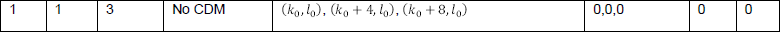k prime = {0} ==> k prime = 0

l prime = {0}  ==> l prime = 0

According to the given, RRC parameters

k0 = 0 (based on frequencyDomainAllocation.row1 = 0001)

l0 = 4(based on firstOFDMSymbolinTimeDomain = 4)

Based on all these information and , we get 38.211-Table 7.4.1.5.3-1,

(k,l) = (k0+kprim,l0+lprim) ,(k0+4+kprim,l0+lprim) ,(k0+8+kprim,l0+lprim)

= (0+0,4+0) ,(0+4+0,4+0) ,(0+8+0,4+0)

= (0,4) ,(4,4) ,(8,4)

 0 1 2 3 4 5 6 7 8 9 10 11 12 13 11 10 9 8 7 6 5 4 3 2 1 0

Example 02 >

Given the following RRC parameters,

density = three

nrofPorts = p1

cdm-Type = noCDM

frequencyDomainAllocation.row1 = 0100

firstOFDMSymbolinTimeDomain = 4

According to 38.211-Table 7.4.1.5.3-1,k prime = {0} ==> k prime = 0

l prime = {0}  ==> l prime = 0

According to the given, RRC parameters

k0 = 2 (based on frequencyDomainAllocation.row1 = 0100)

l0 = 4(based on firstOFDMSymbolinTimeDomain = 4)

Based on all these information and , we get 38.211-Table 7.4.1.5.3-1,

(k,l) = (k0+kprim,l0+lprim) ,(k0+4+kprim,l0+lprim) ,(k0+8+kprim,l0+lprim)

= (2+0,4+0) ,(2+4+0,4+0) ,(2+8+0,4+0)

= (2,4) ,(6,4) ,(10,4)

 0 1 2 3 4 5 6 7 8 9 10 11 12 13 11 10 9 8 7 6 5 4 3 2 1 0

Example 03 >

Given the following RRC parameters, (this example is based on TRS case in 38.508-1 Table 4.6.3-45: CSI-RS-ResourceMapping)

density = three

nrofPorts = p1

cdm-Type = noCDM

frequencyDomainAllocation.row1 = 1000 // From density,nrofPorts we can guess that this is for row1.

firstOFDMSymbolinTimeDomain = 4

According to 38.211-Table 7.4.1.5.3-1,k prime = {0} ==> k prime = 0

l prime = {0}  ==> l prime = 0

According to the given, RRC parameters

k0 = 3 (based on frequencyDomainAllocation.row1 = 1000)

l0 = 4(based on firstOFDMSymbolinTimeDomain = 4)

Based on all these information and , we get 38.211-Table 7.4.1.5.3-1,

(k,l) = (k0+kprim,l0+lprim) ,(k0+4+kprim,l0+lprim) ,(k0+8+kprim,l0+lprim)

= (3+0,4+0) ,(3+4+0,4+0) ,(3+8+0,4+0)

= (3,4) ,(7,4) ,(11,4)

 0 1 2 3 4 5 6 7 8 9 10 11 12 13 11 10 9 8 7 6 5 4 3 2 1 0

Example 04 >

Given the following RRC parameters,

density = one

nrofPorts = p1

cdm-Type = noCDM

frequencyDomainAllocation.row2 = 010000000000

firstOFDMSymbolinTimeDomain = 13

According to 38.211-Table 7.4.1.5.3-1,k prime = {0} ==> k prime = 0

l prime = {0}  ==> l prime = 0

According to the given, RRC parameters

k0 = 10 (based on frequencyDomainAllocation.row2 = 010000000000)

l0 = 13(based on firstOFDMSymbolinTimeDomain = 13)

Based on all these information and , we get 38.211-Table 7.4.1.5.3-1,

(k,l) = (k0+kprim,l0+lprim)

= (10+0,13+0)

= (10,13)

 0 1 2 3 4 5 6 7 8 9 10 11 12 13 11 10 9 8 7 6 5 4 3 2 1 0

Example 05 >

Given the following RRC parameters,

density = one

nrofPorts = p2

cdm-Type = FD-CDM2

frequencyDomainAllocation.other = 001000

firstOFDMSymbolinTimeDomain = 13

Assume that row = 3 (you need additional information to determine the row number other than 1,2,4. In this example, it is given)

According to 38.211-Table 7.4.1.5.3-1,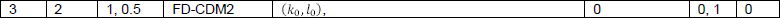k prime = {0,1} ==> k prime = 0, k prime = 1

l prime = {0}  ==> l prime = 0

According to the given, RRC parameters

k0 = 2 x 3(bit position) = 6 (based on frequencyDomainAllocation.other = 001000)

l0 = 13(based on firstOFDMSymbolinTimeDomain = 13)

Based on all these information and , we get 38.211-Table 7.4.1.5.3-1,

(k,l) = (k0+kprim,l0+lprim),(k0+kprim,l0+lprim)

= (6+0,13+0),(6+1,13+0)

= (6,13),(7,13)

 0 1 2 3 4 5 6 7 8 9 10 11 12 13 11 10 9 8 7 wf(1) 6 wf(0) 5 4 3 2 1 0

Example 06 >

Given the following RRC parameters, (this example is based on FR1 case in 38.508-1 Table 4.6.3-45: CSI-RS-ResourceMapping)

density = one

nrofPorts = p8

cdm-Type = fd-CDM2

frequencyDomainAllocation.other = 011110 //From density,nrofPorts,cdm-Tye it is assumed that this is for row6.

firstOFDMSymbolinTimeDomain = 3

According to 38.211-Table 7.4.1.5.3-1,k prime = {0,1} ==> k prime = 0, k prime = 1

l prime = {0}  ==> l prime = 0

According to the given, RRC parameters

k0 = 2 (based on frequencyDomainAllocation.other = 011110)

k1 = 4 (based on frequencyDomainAllocation.other = 011110)

k2 = 6 (based on frequencyDomainAllocation.other = 011110)

k3 = 8 (based on frequencyDomainAllocation.other = 011110)

l0 = 3(based on firstOFDMSymbolinTimeDomain = 3)

Based on all these information and , we get 38.211-Table 7.4.1.5.3-1,

(k,l) = (k0+kprim,l0+lprim) ,(k1+kprim,l0+lprim) ,(k2+kprim,l0+lprim), (k3+kprim,l0+lprim)

(k0+kprim,l0+lprim) ,(k1+kprim,l0+lprim) ,(k2+kprim,l0+lprim), (k3+kprim,l0+lprim)

= (2+0,3+0) ,(4+0,3+0) ,(6+0,3+0),(8+0,3+0),(2+1,3+0) ,(4+1,3+0) ,(6+1,3+0),(8+1,3+0)

= (2,3) ,(4,3) ,(6,3) ,(8,3), (3,3) ,(5,3) ,(7,3) ,(9,3)

 0 1 2 3 4 5 6 7 8 9 10 11 12 13 11 10 9 wf(1) 8 wf(0) 7 wf(1) 6 wf(0) 5 wf(1) 4 wf(0) 3 wf(1) 2 wf(0) 1 0

NOTE : The above resource mapping  is showing the CSI-RS for all antenna ports (8 ports in this case) superimposed in one resource grid. In real transmission, these resources are devided among the 8 CSI-RS antenna ports. How they are splitted among each CSI-RS antenna port is specified in 38.211 section 7.4.1.5.3. Applying the logic in the spec to this example, the antenna port distribution become as follows.

Since this example is using FD-CDM2, it is assumed that 's' is determined by the index value of the following table and 'L' becomes 2. N is given in this example (38.211-Table 7.4.1.5.3-1) is 8.

< 38.211 - Table 7.4.1.5.3-3: The sequences wf(k) f and wt(l) for cdm-Type equal to 'FD-CDM2'. >Based on this information, j and s can be calculated as follows.

s = 0,1 (index of Table 7.4.1.5.3-3)

j = 0,1,...,N/L-1 = 0,1,...,(8/2-1) = 0,1,2,3

Now we can calculate the antenna port as follows.

p = 3000 + s + j L , where s = {0,1}, j = {0,1,2,3}

= 3000 + {0,1} + {0,1,2,3} 2

= {3000, 3002, 3004, 3006, 3001, 3003, 3005, 3007}

NOTE : I posted Matlab simulation for this case showing the resource mapping for each antenna port on this note.

Example 07 >

Given the following RRC parameters, (this example is based on FR1 case in 38.508-1 Table 4.6.3-45: CSI-RS-ResourceMapping)

density = one

nrofPorts = p8

cdm-Type = fd-CDM2

frequencyDomainAllocation.other = 000110 //From density,nrofPorts,cdm-Tye it is assumed that this is for row7.

firstOFDMSymbolinTimeDomain = 3

According to 38.211-Table 7.4.1.5.3-1,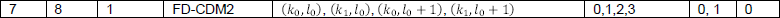k prime = {0,1} ==> k prime = 0, k prime = 1

l prime = {0}  ==> l prime = 0

According to the given, RRC parameters

k0 = 2 (based on frequencyDomainAllocation.other = 000110)

k1 = 4 (based on frequencyDomainAllocation.other = 000110)

l0 = 3(based on firstOFDMSymbolinTimeDomain = 3)

Based on all these information and , we get 38.211-Table 7.4.1.5.3-1,

(k,l) = (k0+kprim,l0+lprim) ,(k1+kprim,l0+lprim) ,

(k0+kprim,l0+lprim+1), (k1+kprim,l0+lprim+1)

(k0+kprim,l0+lprim) ,(k1+kprim,l0+lprim) ,

(k0+kprim,l0+lprim+1), (k1+kprim,l0+lprim+1)

= (2+0,3+0) ,(4+0,3+0) ,(2+0,3+0+1) ,(4+0,3+0+1),(2+1,3+0) ,(4+1,3+0) ,(2+1,3+0+1) ,(4+1,3+0+1)

= (2,3) ,(4,3) ,(2,4),(4,4),(3,3),(5,3),(3,4),(5,4)

 0 1 2 3 4 5 6 7 8 9 10 11 12 13 11 10 9 8 7 6 5 wf(1) wf(1) 4 wf(0) wf(0) 3 wf(1) wf(1) 2 wf(0) wf(0) 1 0

NOTE : The above resource mapping  is showing the CSI-RS for all antenna ports (8 ports in this case) superimposed in one resource grid. In real transmission, these resources are devided among the 8 CSI-RS antenna ports. How they are splitted among each CSI-RS antenna port is specified in 38.211 section 7.4.1.5.3. Applying the logic in the spec to this example, the antenna port distribution become as follows.

Since this example is using FD-CDM2, it is assumed that 's' is determined by the index value of the following table and 'L' becomes 2. N is given in this example (38.211-Table 7.4.1.5.3-1) is 8.

< 38.211 - Table 7.4.1.5.3-3: The sequences wf(k) f and wt(l) for cdm-Type equal to 'FD-CDM2'. >Based on this information, j and s can be calculated as follows.

s = 0,1 (index of Table 7.4.1.5.3-3)

j = 0,1,...,N/L-1 = 0,1,...,(8/2-1) = 0,1,2,3

Now we can calculate the antenna port as follows.

p = 3000 + s + j L , where s = {0,1}, j = {0,1,2,3}

= 3000 + {0,1} + {0,1,2,3} 2

= {3000, 3002, 3004, 3006, 3001, 3003, 3005, 3007}

NOTE : I posted Matlab simulation for this case showing the resource mapping for each antenna port on this note.

Example 08 >

Given the following RRC parameters, (this example is based on FR1 case in 38.508-1 Table 4.6.3-45: CSI-RS-ResourceMapping)

density = one

nrofPorts = p8

cdm-Type = CDM4(FD2,TD2)

frequencyDomainAllocation.other = 000110 //From density,nrofPorts,cdm-Tye it is assumed that this is for row8.

firstOFDMSymbolinTimeDomain = 3

According to 38.211-Table 7.4.1.5.3-1,k prime = {0,1} ==> k prime = 0, k prime = 1

l prime = {0,1}  ==> l prime = 0, l prime = 1

According to the given, RRC parameters

k0 = 2 (based on frequencyDomainAllocation.other = 000110)

k1 = 4 (based on frequencyDomainAllocation.other = 000110)

l0 = 3(based on firstOFDMSymbolinTimeDomain = 3)

Based on all these information and , we get 38.211-Table 7.4.1.5.3-1,

(k,l) = (k0+kprim,l0+lprim) ,(k1+kprim,l0+lprim),

(k0+kprim,l0+lprim) ,(k1+kprim,l0+lprim),

(k0+kprim,l0+lprim) ,(k1+kprim,l0+lprim),

(k0+kprim,l0+lprim) ,(k1+kprim,l0+lprim),

= (2+0,3+0) ,(4+0,3+0) ,(2+1,3+0) ,(4+1,3+0),(2+0,3+1) ,(4+0,3+1) ,(2+1,3+1) ,(4+1,3+1)

= (2,3) ,(4,3) ,(3,3),(5,3),(2,4),(4,4),(3,4),(5,4)

 0 1 2 3 4 5 6 7 8 9 10 11 12 13 11 10 9 8 7 6 5 wft1 wft1 4 wft0 wft0 3 wft1 wft1 2 wft0 wft0 1 0

NOTE : wft0 = wf(0) wt(0), wft1 = wf(1) wt(1)

NOTE : The above resource mapping  is showing the CSI-RS for all antenna ports (8 ports in this case) superimposed in one resource grid. In real transmission, these resources are devided among the 8 CSI-RS antenna ports. How they are splitted among each CSI-RS antenna port is specified in 38.211 section 7.4.1.5.3. Applying the logic in the spec to this example, the antenna port distribution become as follows.

Since this example is using FD-CDM2, it is assumed that 's' is determined by the index value of the following table and 'L' becomes 4. N is given in this example (38.211-Table 7.4.1.5.3-1) is 8.

< 38.211 - Table 7.4.1.5.3-4: The sequences wf(k) f and wt(l) for cdm-Type equal to 'CDM4'. >Based on this information, j and s can be calculated as follows.

s = 0,1,2,3 (index of Table 7.4.1.5.3-4)

j = 0,1,...,N/L-1 = 0,1,...,(8/4-1) = 0,1

Now we can calculate the antenna port as follows.

p = 3000 + s + j L , where s = {0,1}, j = {0,1,2,3}

= 3000 + {0,1,2,3} + {0,1} 4

= {3000, 3004, 3004, 3006, 3001, 3003, 3005, 3007}

NOTE : I posted Matlab simulation for this case showing the resource mapping for each antenna port on this note.

CSI RS Transmission Timing

CSI Transmission Timing in slot is determined by RRC parameter CSI-ResourcePeriodicityAndOffset based on following equation.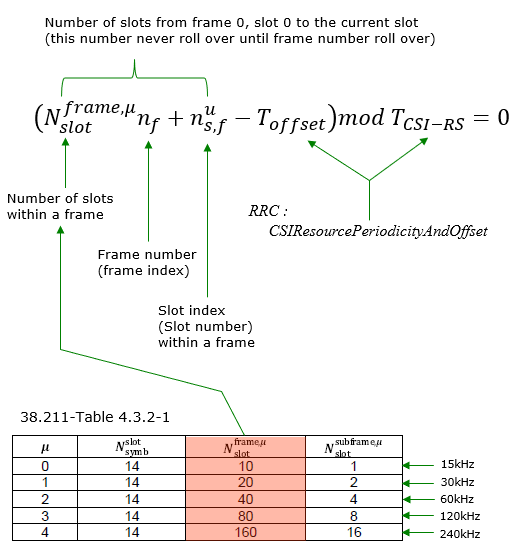CSI IM resource is a set of specific resource elements reserved for Interference Measurement. This resources are configurable by RRC message. The frequency and time domain location is defined in 38.214 - 5.2.2.4 as illustrated below.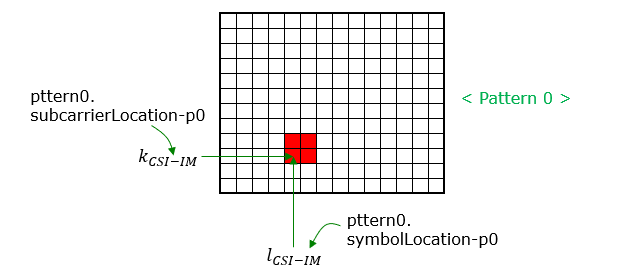CSI-IM-Resource ::= SEQUENCE {

csi-IM-ResourceId                   CSI-IM-ResourceId,

csi-IM-ResourceElementPattern       CHOICE {

pattern0 SEQUENCE {

subcarrierLocation-p0         ENUMERATED { s0, s2, s4, s6, s8, s10 },

symbolLocation-p0             INTEGER (0..12)

},

pattern1 SEQUENCE {

subcarrierLocation-p1         ENUMERATED { s0, s4, s8 },

symbolLocation-p1             INTEGER (0..13)

}

} OPTIONAL, -- Need M

freqBand                            CSI-FrequencyOccupation OPTIONAL,

periodicityAndOffset                CSI-ResourcePeriodicityAndOffset OPTIONAL,

PeriodicOrSemiPersistent

...

}

pattern 0 / pattern1 : illustrated above.

CSI-FrequencyOccupation ::= SEQUENCE {

startingRB    INTEGER (0..maxNrofPhysicalResourceBlocks-1),

nrofRBs       INTEGER (24..maxNrofPhysicalResourceBlocksPlus1),

...

}

startingRB : PRB where this CSI resource starts in relation to common resource block #0 (CRB#0) on the common resource block grid. Only multiples of 4 are allowed (0, 4, ...)

nrofRBs : Number of PRBs across which this CSI resource spans. Only multiples of 4 are allowed. The smallest configurable number is the minimum of 24 and the width of the associated BWP. If the configured value is larger than the width of the corresponding BWP, the UE shall assume that the actual CSI-RS bandwidth is equal to the width of the BWP.

Tracking Reference Signal (TRS)

NZP-CSI-RS-ResourceSet ::= SEQUENCE {

nzp-CSI-ResourceSetId     NZP-CSI-RS-ResourceSetId,

nzp-CSI-RS-Resources      SEQUENCE (SIZE (1..maxNrofNZP-CSI-RS-ResourcesPerSet))

OF NZP-CSI-RS-ResourceId,

repetition                ENUMERATED { on, off } OPTIONAL,

aperiodicTriggeringOffset INTEGER(0..4) OPTIONAL,

trs-Info                  ENUMERATED {true} OPTIONAL,

...

}

38.331 defines trs-Info as follows.

trs-Info : Indicates that the antenna port for all NZP-CSI-RS resources in the CSI-RS resource set is same. If the field is absent or released the UE applies the value false

38.214-5.2.2.3.1 provides additional information as follows :

trs-Info in NZP-CSI-RS-ResourceSet is associated with a CSI-RS resource set and for which the UE can assume that the antenna port with the same port index of the configured NZP CSI-RS resources in the NZP-CSI-RSResourceSet is the same as described in Subclause 5.1.6.1.1 and can be configured when reporting setting is not configured or when the higher layer parameter reportQuantity associated with all the reporting settings linked with the CSI-RS resource set is set to 'none'.

38.214-5.1.6.1.1 specifies the condition about how multiple csi-rs is grouped into a trs as stated below.

For a NZP-CSI-RS-ResourceSet configured with the higher layer parameter trs-Info, the UE shall assume the antenna

port with the same port index of the configured NZP CSI-RS resources in the NZP-CSI-RS-ResourceSet is the same. For frequency range 1(FR1), the UE may be configured with one or more NZP CSI-RS set(s), where a NZP-CSI-RS-ResourceSet consists of four periodic NZP CSI-RS resources in two consecutive slots with two periodic NZP CSI-RS resources in each slot.

For frequency range 2(FR2) the UE may be configured with one or more NZP CSI-RS set(s), where a NZP-CSI-RSResourceSet consists of two periodic CSI-RS resources in one slot or with a NZP-CSI-RS-ResourceSet of four periodic NZP CSI-RS resources in two consecutive slots with two periodic NZP CSI-RS resources in each slot.

NOTE : trs-info setting affects the applicable QCL type which is described in this note.

Zero Power CSI-RS

Zero Power CSI-RS is not really transmitting any signal, it is designed for make a blank RE in the PDSCH resource grid. Why we need this ?  The answer is well described in VI-C of this paper as follows:

Zero-power CSI-RS can be used as a masking tool to protect certain REs by making them unavailable for PDSCH mapping. This masking supports transmission of UE specific CSI-RS, but the design is also a tool for allowing introduction of new features to NR with retained backward compatibility

RRC Parameters for CSI RS

The hierarchy to configure CSI-RS is pretty complicated. Overall hierachy (procedure) to configure CSI-RS can be described as follows.

PDSCH-Config ::=                        SEQUENCE {

dataScramblingIdentityPDSCH             INTEGER (0..1007)    OPTIONAL,

OF TCI-State    OPTIONAL,   -- Need N

tci-StatesToReleaseList                 SEQUENCE (SIZE(1..maxNrofTCI-States))

OF TCI-StateId  OPTIONAL,   -- Need N

vrb-ToPRB-Interleaver                   ENUMERATED {n2, n4},

resourceAllocation                      ENUMERATED { resourceAllocationType0,

resourceAllocationType1,

dynamicSwitch},

pdsch-AllocationList                    SEQUENCE (SIZE(1..maxNrofDL-Allocations))

OF PDSCH-TimeDomainResourceAllocation ,

pdsch-AggregationFactor                 ENUMERATED { n2, n4, n8 } OPTIONAL,

rateMatchPatternToAddModList            SEQUENCE (SIZE (1..maxNrofRateMatchPatterns))

OF RateMatchPattern    OPTIONAL, -- Need N

rateMatchPatternToReleaseList           SEQUENCE (SIZE (1..maxNrofRateMatchPatterns))

OF RateMatchPatternId     OPTIONAL, -- Need N

rateMatchPatternGroup1                  SEQUENCE (SIZE (1..maxNrofRateMatchPatterns))

OF RateMatchPatternId     OPTIONAL, -- Need R

rateMatchPatternGroup2                  SEQUENCE (SIZE (1..maxNrofRateMatchPatterns))

OF RateMatchPatternId     OPTIONAL, -- Need R

rbg-Size                                ENUMERATED {config1, config2},

mcs-Table                               ENUMERATED {qam64, qam256},

maxNrofCodeWordsScheduledByDCI          ENUMERATED {n1, n2} OPTIONAL,   -- Need R

prb-BundlingType                    CHOICE {

static                                  SEQUENCE {

bundleSize                              ENUMERATED { n4, wideband }  OPTIONAL

},

dynamic                                 SEQUENCE {

bundleSizeSet1                          ENUMERATED { n4,

wideband,

n2-wideband,

n4-wideband

}  OPTIONAL,   -- Need S

bundleSizeSet2                          ENUMERATED { n4,

wideband

} OPTIONAL    -- Need S

}

},

zp-CSI-RS-ResourceToAddModList          SEQUENCE (SIZE (1..maxNrofZP-CSI-RS-Resources))

OF ZP-CSI-RS-Resource   OPTIONAL,   -- Need N

zp-CSI-RS-ResourceToReleaseList         SEQUENCE (SIZE (1..maxNrofZP-CSI-RS-Resources))

OF ZP-CSI-RS-ResourceId OPTIONAL,   -- Need M

aperiodic-ZP-CSI-RS-ResourceSetsToAddModList    SEQUENCE (SIZE (1..maxNrofZP-CSI-RS-Sets))

OF ZP-CSI-RS-ResourceSet   OPTIONAL, -- Need N

aperiodic-ZP-CSI-RS-ResourceSetsToReleaseList   SEQUENCE (SIZE (1..maxNrofZP-CSI-RS-Sets))

OF ZP-CSI-RS-ResourceSetId   OPTIONAL, -- Need N

sp-ZP-CSI-RS-ResourceSetsToAddModList   SEQUENCE (SIZE (1..maxNrofZP-CSI-RS-Sets))

OF ZP-CSI-RS-ResourceSet     OPTIONAL, -- Need N

sp-ZP-CSI-RS-ResourceSetsToReleaseList  SEQUENCE (SIZE (1..maxNrofZP-CSI-RS-Sets))

OF ZP-CSI-RS-ResourceSetId   OPTIONAL, -- Need N

...

}

CSI-MeasConfig ::= SEQUENCE {

nzp-CSI-RS-ResourceToAddModList          SEQUENCE (SIZE (1..maxNrofNZP-CSI-RS-Resources))

OF NZP-CSI-RS-Resource OPTIONAL,

nzp-CSI-RS-ResourceToReleaseList         SEQUENCE (SIZE (1..maxNrofNZP-CSI-RS-Resources))

OF NZP-CSI-RS-ResourceId OPTIONAL,

nzp-CSI-RS-ResourceSetToAddModList       SEQUENCE (SIZE (1..maxNrofNZP-CSI-RS-ResourceSets))

OF NZP-CSI-RS-ResourceSet OPTIONAL,

nzp-CSI-RS-ResourceSetToReleaseList      SEQUENCE (SIZE (1..maxNrofNZP-CSI-RS-ResourceSets))

OF NZP-CSI-RS-ResourceSetId OPTIONAL,

csi-IM-ResourceToAddModList              SEQUENCE (SIZE (1..maxNrofCSI-IM-Resources))

OF CSI-IM-Resource OPTIONAL,

csi-IM-ResourceToReleaseList             SEQUENCE (SIZE (1..maxNrofCSI-IM-Resources))

OF CSI-IM-ResourceId OPTIONAL,

csi-IM-ResourceSetToAddModList           SEQUENCE (SIZE (1..maxNrofCSI-IM-ResourceSets))

OF CSI-IM-ResourceSet OPTIONAL,

csi-IM-ResourceSetToReleaseList          SEQUENCE (SIZE (1..maxNrofCSI-IM-ResourceSets))

OF CSI-IM-ResourceSetId OPTIONAL,

csi-SSB-ResourceSetToAddModList          SEQUENCE (SIZE (1..maxNrofCSI-SSB-ResourceSets))

OF CSI-SSB-ResourceSet OPTIONAL,

csi-SSB-ResourceSetToAddReleaseList      SEQUENCE (SIZE (1..maxNrofCSI-SSB-ResourceSets))

OF CSI-SSB-ResourceSetId OPTIONAL,

csi-ResourceConfigToAddModList           SEQUENCE (SIZE (1..maxNrofCSI-ResourceConfigurations))

OF CSI-ResourceConfig OPTIONAL,

csi-ResourceConfigToReleaseList          SEQUENCE (SIZE (1..maxNrofCSI-ResourceConfigurations))

OF CSI-ResourceConfigId OPTIONAL,

csi-ReportConfigToAddModList             SEQUENCE (SIZE (1..maxNrofCSI-ReportConfigurations))

OF CSI-ReportConfig OPTIONAL,

csi-ReportConfigToReleaseList            SEQUENCE (SIZE (1..maxNrofCSI-ReportConfigurations))

OF CSI-ReportConfigId OPTIONAL,

reportTriggerSize                        INTEGER (0..6) OPTIONAL,

aperiodicTriggerStateList                SetupRelease { CSI-AperiodicTriggerStateList },

semiPersistentOnPUSCH-TriggerStateList   SetupRelease {

CSI-SemiPersistentOnPUSCH-TriggerStateList

} OPTIONAL,

...

}

ZP-CSI-RS-Resource ::= SEQUENCE {

zp-CSI-RS-ResourceId        ZP-CSI-RS-ResourceId,

resourceMapping             CSI-RS-ResourceMapping,

periodicityAndOffset        CSI-ResourcePeriodicityAndOffset OPTIONAL,

...

}

ZP-CSI-RS-ResourceSet ::= SEQUENCE {

zp-CSI-RS-ResourceSetId    ZP-CSI-RS-ResourceSetId,

zp-CSI-RS-ResourceIdList   SEQUENCE (SIZE(1..maxNrofZP-CSI-RS-ResourcesPerSet))

OF ZP-CSI-RS-ResourceId,

...

}

NZP-CSI-RS-Resource ::= SEQUENCE {

nzp-CSI-RS-ResourceId      NZP-CSI-RS-ResourceId,

resourceMapping            CSI-RS-ResourceMapping,

powerControlOffset         INTEGER (-8..15),

powerControlOffsetSS       ENUMERATED{db-3, db0, db3, db6} OPTIONAL, -- Need R

scramblingID               ScramblingId,

periodicityAndOffset       CSI-ResourcePeriodicityAndOffset OPTIONAL,-

qcl-InfoPeriodicCSI-RS     TCI-StateId OPTIONAL, -- Cond Periodic

...

}

NZP-CSI-RS-ResourceSet ::= SEQUENCE {

nzp-CSI-ResourceSetId     NZP-CSI-RS-ResourceSetId,

nzp-CSI-RS-Resources      SEQUENCE (SIZE (1..maxNrofNZP-CSI-RS-ResourcesPerSet))

OF NZP-CSI-RS-ResourceId,

repetition                ENUMERATED { on, off } OPTIONAL,

aperiodicTriggeringOffset INTEGER(0..4) OPTIONAL,

trs-Info                  ENUMERATED {true} OPTIONAL,

...

}

CSI-RS-ResourceMapping ::= SEQUENCE {

frequencyDomainAllocation CHOICE {

row1     BIT STRING (SIZE (4)),

row2     BIT STRING (SIZE (12)),

row4     BIT STRING (SIZE (3)),

other    BIT STRING (SIZE (6))

},

nrofPorts                       ENUMERATED {p1,p2,p4,p8,p12,p16,p24,p32},

firstOFDMSymbolInTimeDomain     INTEGER (0..13),

firstOFDMSymbolInTimeDomain2    INTEGER (2..12) OPTIONAL, -- Need R

cdm-Type                        ENUMERATED {noCDM, fd-CDM2, cdm4-FD2-TD2, cdm8-FD2-TD4},

density CHOICE {

dot5      ENUMERATED {evenPRBs, oddPRBs},

one       NULL,

three     NULL,

spare     NULL

},

freqBand    CSI-FrequencyOccupation,

...

}

CSI-ResourcePeriodicityAndOffset ::= CHOICE {

slots4       INTEGER (0..3),

slots5       INTEGER (0..4),

slots8       INTEGER (0..7),

slots10      INTEGER (0..9),

slots16      INTEGER (0..15),

slots20      INTEGER (0..19),

slots32      INTEGER (0..31),

slots40      INTEGER (0..39),

slots64      INTEGER (0..63),

slots80      INTEGER (0..79),

slots160     INTEGER (0..159),

slots320     INTEGER (0..319),

slots640     INTEGER (0..639)

}

CSI-FrequencyOccupation ::= SEQUENCE {

startingRB    INTEGER (0..maxNrofPhysicalResourceBlocks-1),

nrofRBs       INTEGER (24..maxNrofPhysicalResourceBlocksPlus1),

...

}

CSI-IM-Resource ::= SEQUENCE {

csi-IM-ResourceId                   CSI-IM-ResourceId,

csi-IM-ResourceElementPattern       CHOICE {

pattern0 SEQUENCE {

subcarrierLocation-p0         ENUMERATED { s0, s2, s4, s6, s8, s10 },

symbolLocation-p0             INTEGER (0..12)

},

pattern1 SEQUENCE {

subcarrierLocation-p1         ENUMERATED { s0, s4, s8 },

symbolLocation-p1             INTEGER (0..13)

}

} OPTIONAL, -- Need M

freqBand                            CSI-FrequencyOccupation OPTIONAL,

periodicityAndOffset                CSI-ResourcePeriodicityAndOffset OPTIONAL,

PeriodicOrSemiPersistent

...

}

CSI-IM-ResourceSet ::= SEQUENCE {

csi-IM-ResourceSetId              CSI-IM-ResourceSetId,

csi-IM-Resources                  SEQUENCE (SIZE(1..maxNrofCSI-IM-ResourcesPerSet))

OF CSI-IM-ResourceId,

...

}

CSI-SSB-ResourceSet ::= SEQUENCE {

csi-SSB-ResourceSetId            CSI-SSB-ResourceSetId,

csi-SSB-ResourceList             SEQUENCE (SIZE(1..maxNrofCSI-SSB-ResourcePerSet))

OF SSB-Index,

...

}

CSI-ResourceConfig ::= SEQUENCE {

csi-ResourceConfigId           CSI-ResourceConfigId,

csi-RS-ResourceSetList         CHOICE {

nzp-CSI-RS-SSB                 SEQUENCE {

nzp-CSI-RS-ResourceSetList   SEQUENCE (SIZE (1..maxNrofNZP-CSI-RS-ResourceSetsPerConfig))

OF NZP-CSI-RS-ResourceSetId OPTIONAL,

csi-SSB-ResourceSetList      SEQUENCE (SIZE (1..maxNrofCSI-SSB-ResourceSetsPerConfig))

OF CSI-SSB-ResourceSetId OPTIONAL

},

csi-IM-ResourceSetList          SEQUENCE (SIZE (1..maxNrofCSI-IM-ResourceSetsPerConfig))

OF CSI-IM-ResourceSetId

},

bwp-Id                         BWP-Id,

resourceType                   ENUMERATED { aperiodic, semiPersistent, periodic },

...

}

CSI-ReportConfig ::= SEQUENCE {

reportConfigId                         CSI-ReportConfigId,

carrier                                ServCellIndex OPTIONAL,

resourcesForChannelMeasurement         CSI-ResourceConfigId,

csi-IM-ResourcesForInterference        CSI-ResourceConfigId OPTIONAL,

nzp-CSI-RS-ResourcesForInterference    CSI-ResourceConfigId OPTIONAL,

reportConfigType                       CHOICE {

periodic SEQUENCE {

reportSlotConfig                 CSI-ReportPeriodicityAndOffset,

pucch-CSI-ResourceList           SEQUENCE (SIZE (1..maxNrofBWPs)) OF PUCCH-CSI-Resource

},

semiPersistentOnPUCCH SEQUENCE {

reportSlotConfig                 CSI-ReportPeriodicityAndOffset,

pucch-CSI-ResourceList           SEQUENCE (SIZE (1..maxNrofBWPs)) OF PUCCH-CSI-Resource

},

semiPersistentOnPUSCH SEQUENCE {

reportSlotConfig                 ENUMERATED {sl5, sl10, sl20, sl40, sl80, sl160, sl320},

reportSlotOffsetList             SEQUENCE (SIZE (1.. maxNrofUL-Allocations))

OF INTEGER(0..32),

p0alpha                             P0-PUSCH-AlphaSetId

},

aperiodic SEQUENCE {

reportSlotOffsetList             SEQUENCE (SIZE (1..maxNrofUL-Allocations))

OF INTEGER(0..32)

}

},

reportQuantity CHOICE {

none NULL,

cri-RI-PMI-CQI                      NULL,

cri-RI-i1                           NULL,

cri-RI-i1-CQI                       SEQUENCE {

pdsch-BundleSizeForCSI                ENUMERATED {n2, n4} OPTIONAL

},

cri-RI-CQI                          NULL,

cri-RSRP                            NULL,

ssb-Index-RSRP                      NULL,

cri-RI-LI-PMI-CQI                   NULL

},

reportFreqConfiguration SEQUENCE {

cqi-FormatIndicator                 ENUMERATED { widebandCQI, subbandCQI } OPTIONAL,

pmi-FormatIndicator                 ENUMERATED { widebandPMI, subbandPMI } OPTIONAL,

csi-ReportingBand CHOICE {

subbands3                        BIT STRING(SIZE(3)),

subbands4                        BIT STRING(SIZE(4)),

subbands5                        BIT STRING(SIZE(5)),

subbands6                        BIT STRING(SIZE(6)),

subbands7                        BIT STRING(SIZE(7)),

subbands8                        BIT STRING(SIZE(8)),

subbands9                        BIT STRING(SIZE(9)),

subbands10                       BIT STRING(SIZE(10)),

subbands11                       BIT STRING(SIZE(11)),

subbands12                       BIT STRING(SIZE(12)),

subbands13                       BIT STRING(SIZE(13)),

subbands14                       BIT STRING(SIZE(14)),

subbands15                       BIT STRING(SIZE(15)),

subbands16                       BIT STRING(SIZE(16)),

subbands17                       BIT STRING(SIZE(17)),

subbands18                       BIT STRING(SIZE(18)),

...,

subbands19-v1530                 BIT STRING(SIZE(19))

} OPTIONAL

} OPTIONAL,

timeRestrictionForChannelMeasurements          ENUMERATED {configured, notConfigured},

timeRestrictionForInterferenceMeasurements     ENUMERATED {configured, notConfigured},

codebookConfig                                 CodebookConfig OPTIONAL,

nrofCQIsPerReport                              ENUMERATED {n1, n2} OPTIONAL,

groupBasedBeamReporting                CHOICE {

enabled                                 NULL,

disabled                            SEQUENCE {

nrofReportedRS                          ENUMERATED {n1, n2, n3, n4} OPTIONAL

}

},

cqi-Table                              ENUMERATED {table1, table2, table3, spare1} OPTIONAL,

subbandSize                            ENUMERATED {value1, value2},

non-PMI-PortIndication                 SEQUENCE (SIZE (1..maxNrofNZP-CSI-RS-ResourcesPerConfig))

OF PortIndexFor8Ranks OPTIONAL,

...,

[[

semiPersistentOnPUSCH-v1530 SEQUENCE {

reportSlotConfig-v1530             ENUMERATED {sl4, sl8, sl16}

} OPTIONAL

]]

}

CSI-ReportPeriodicityAndOffset ::= CHOICE {

slots4          INTEGER(0..3),

slots5          INTEGER(0..4),

slots8          INTEGER(0..7),

slots10         INTEGER(0..9),

slots16         INTEGER(0..15),

slots20         INTEGER(0..19),

slots40         INTEGER(0..39),

slots80         INTEGER(0..79),

slots160        INTEGER(0..159),

slots320        INTEGER(0..319)

}

PUCCH-CSI-Resource ::= SEQUENCE {

pucch-Resource                PUCCH-ResourceId

}

PortIndexFor8Ranks ::= CHOICE {

portIndex8    SEQUENCE{

rank1-8         PortIndex8 OPTIONAL, -- Need R

rank2-8         SEQUENCE(SIZE(2)) OF PortIndex8 OPTIONAL, -- Need R

rank3-8         SEQUENCE(SIZE(3)) OF PortIndex8 OPTIONAL, -- Need R

rank4-8         SEQUENCE(SIZE(4)) OF PortIndex8 OPTIONAL, -- Need R

rank5-8         SEQUENCE(SIZE(5)) OF PortIndex8 OPTIONAL, -- Need R

rank6-8         SEQUENCE(SIZE(6)) OF PortIndex8 OPTIONAL, -- Need R

rank7-8         SEQUENCE(SIZE(7)) OF PortIndex8 OPTIONAL, -- Need R

rank8-8         SEQUENCE(SIZE(8)) OF PortIndex8 OPTIONAL -- Need R

},

portIndex4    SEQUENCE{

rank1-4         PortIndex4 OPTIONAL, -- Need R

rank2-4         SEQUENCE(SIZE(2)) OF PortIndex4 OPTIONAL, -- Need R

rank3-4         SEQUENCE(SIZE(3)) OF PortIndex4 OPTIONAL, -- Need R

rank4-4         SEQUENCE(SIZE(4)) OF PortIndex4 OPTIONAL -- Need R

},

portIndex2    SEQUENCE{

rank1-2         PortIndex2 OPTIONAL, -- Need R

rank2-2         SEQUENCE(SIZE(2)) OF PortIndex2 OPTIONAL -- Need R

},

portIndex1         NULL

}

CodebookConfig ::= SEQUENCE {

codebookType CHOICE {

type1 SEQUENCE {

subType CHOICE {

typeI-SinglePanel SEQUENCE {

nrOfAntennaPorts CHOICE {

two SEQUENCE {

twoTX-CodebookSubsetRestriction BIT STRING (SIZE (6))

},

moreThanTwo SEQUENCE {

n1-n2 CHOICE {

two-one-TypeI-SinglePanel-Restriction        BIT STRING (SIZE (8)),

two-two-TypeI-SinglePanel-Restriction        BIT STRING (SIZE (64)),

four-one-TypeI-SinglePanel-Restriction       BIT STRING (SIZE (16)),

three-two-TypeI-SinglePanel-Restriction      BIT STRING (SIZE (96)),

six-one-TypeI-SinglePanel-Restriction        BIT STRING (SIZE (24)),

four-two-TypeI-SinglePanel-Restriction       BIT STRING (SIZE (128)),

eight-one-TypeI-SinglePanel-Restriction      BIT STRING (SIZE (32)),

four-three-TypeI-SinglePanel-Restriction     BIT STRING (SIZE (192)),

six-two-TypeI-SinglePanel-Restriction        BIT STRING (SIZE (192)),

twelve-one-TypeI-SinglePanel-Restriction     BIT STRING (SIZE (48)),

four-four-TypeI-SinglePanel-Restriction      BIT STRING (SIZE (256)),

eight-two-TypeI-SinglePanel-Restriction      BIT STRING (SIZE (256)),

sixteen-one-TypeI-SinglePanel-Restriction    BIT STRING (SIZE (64))

},

typeI-SinglePanel-codebookSubsetRestriction-i2     BIT STRING (SIZE (16))

}

},

typeI-SinglePanel-ri-Restriction BIT STRING (SIZE (8))

},

typeI-MultiPanel SEQUENCE {

ng-n1-n2 CHOICE {

two-two-one-TypeI-MultiPanel-Restriction          BIT STRING (SIZE (8)),

two-four-one-TypeI-MultiPanel-Restriction         BIT STRING (SIZE (16)),

four-two-one-TypeI-MultiPanel-Restriction         BIT STRING (SIZE (8)),

two-two-two-TypeI-MultiPanel-Restriction          BIT STRING (SIZE (64)),

two-eight-one-TypeI-MultiPanel-Restriction        BIT STRING (SIZE (32)),

four-four-one-TypeI-MultiPanel-Restriction        BIT STRING (SIZE (16)),

two-four-two-TypeI-MultiPanel-Restriction         BIT STRING (SIZE (128)),

four-two-two-TypeI-MultiPanel-Restriction         BIT STRING (SIZE (64))

},

ri-Restriction BIT STRING (SIZE (4))

}

},

codebookMode INTEGER (1..2)

},

type2 SEQUENCE {

subType CHOICE {

typeII SEQUENCE {

n1-n2-codebookSubsetRestriction CHOICE {

two-one               BIT STRING (SIZE (16)),

two-two               BIT STRING (SIZE (43)),

four-one              BIT STRING (SIZE (32)),

three-two             BIT STRING (SIZE (59)),

six-one               BIT STRING (SIZE (48)),

four-two              BIT STRING (SIZE (75)),

eight-one             BIT STRING (SIZE (64)),

four-three            BIT STRING (SIZE (107)),

six-two               BIT STRING (SIZE (107)),

twelve-one            BIT STRING (SIZE (96)),

four-four             BIT STRING (SIZE (139)),

eight-two             BIT STRING (SIZE (139)),

sixteen-one           BIT STRING (SIZE (128))

},

typeII-RI-Restriction    BIT STRING (SIZE (2))

},

typeII-PortSelection SEQUENCE {

portSelectionSamplingSize              ENUMERATED {n1, n2, n3, n4} OPTIONAL,

typeII-PortSelectionRI-Restriction     BIT STRING (SIZE (2))

}

},

phaseAlphabetSize                            ENUMERATED {n4, n8},

subbandAmplitude                             BOOLEAN,

numberOfBeams                                ENUMERATED {two, three, four}

}

}

}

RRC Examples

Following is a simple example of CSI-RS allocation from 38.508-1.  You would have more details configurations from the same specification that I summarized in this note.

< Based on 38.508-1 Table 4.6.3-45: CSI-RS-ResourceMapping >

 TRS FR1 FR2 CSI-RS-ResourceMapping ::= SEQUENCE {   frequencyDomainAllocation CHOICE   { row1 1000 row4 010 other 011110 } nrofPorts p1 p8 p4 firstOFDMSymbolInTimeDomain 4 3 13 firstOFDMSymbolInTimeDomain2 Not present cdm-Type noCDM fd-CDM2 fd-CDM2 density CHOICE { one three NULL } freqBand CSI-FrequencyOccupation } NOTE see here for CSI-RS map see here for CSI-RS map

< Based on 38.508-1 Table 4.6.3-33: CSI-FrequencyOccupation >

 FR1_60 FR1_80 FR1_100 FR2_100 TRS CSI-FrequencyOccupation ::= SEQUENCE { startingRB 0 0 0 0 0 nrofRBs 160 216 272 64 52 }

How to Avoid Collision with Other Signals ?

According to what is explained above, it would be possible to allocate the CSI-RS at any symbol and in any slot, but in real situation where various other physical channels and signals running we cannot enjoy such a full degree of freedom for allocating CSI-RS.  In this aspect, I think I can list some tips that may helps.

• Avoid Symbols where SSB is transmitted
• Avoid slots and symbols that are configured for Uplink in TDD UL-DL configuration
• Avoid symbols where CORESET is configured
• Avoid symbols where PDSCH DMRS is configured

Example 1 >

As an example, let's assume a case as follows.

SIB 1 :

ssb-PositionsInBurst {

inOneGroup 'FF'H

},

ssb-PeriodicityServingCell ms20,

tdd-UL-DL-ConfigurationCommon {

referenceSubcarrierSpacing kHz30,

pattern1 {

dl-UL-TransmissionPeriodicity ms5,

}

},

First thing I want to suggest you to do is to draw a diagram and mark the configuration of ssb bitmap and tdd-UL-DL config and some additional symbols where CSI-RS is not allowed to be assigned as below.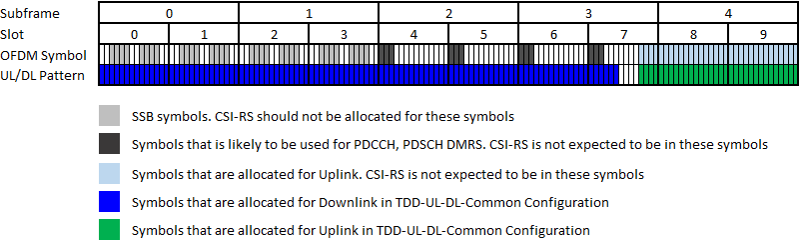Now you have a bunck of white spaces where you can allocate CSI-RS. Not so many white spaces, right ? Actually if you want to put the CSI-RS to another 5ms period where SSB is not transmitted, it would be much easier to configure, but in this example I intentionaly picked up tough situation. There can be so many different ways of configuring the CSI-RS, but you can determine any valid configuration as you want and mark it as shown below (this is just one example) and then populate the configuration into RRC message. It would be very error prone if you try configuring RRC without this kind of drawing beforehand.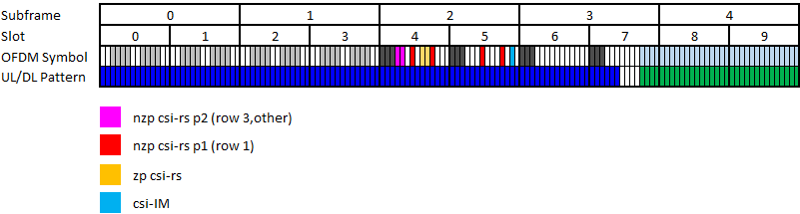NOTE : I have tested this configuration with Amarisoft Callbox (gNB+Core) and two different UE (Amarisoft UE simulator and a commericial UE).

Case 1 : I configured CSI for TRS at the different symbol number in slot 4 and 5. With this configuration, only Amarisoft UE simulator passed and commercial UE didn't pass (Radio Link broken right after RRC Setup).

Case 2 : I configured CSI for TRS at the same symbol number in slot 4 and 5 then both DUT (Amarisoft UEsimulator and Commerical UE) passed. I think both configuration is compliant to 3GPP and I personally think it is due to UE implementation of the commercial UE that failed at case 1).

Reference